# Gradient Descent Methods¶

This tour explores the use of gradient descent method for unconstrained and constrained optimization of a smooth function

Important: Please read the installation page for details about how to install the toolboxes. $\newcommand{\dotp}{\langle #1, #2 \rangle}$ $\newcommand{\pd}{ \frac{ \partial #1}{\partial #2} }$ $\newcommand{\umin}{\underset{#1}{\min}\;}$ $\newcommand{\qandq}{\quad\text{and}\quad}$ $\newcommand{\qwhereq}{\quad\text{where}\quad}$ $\newcommand{\qifq}{ \quad \text{if} \quad }$ $\newcommand{\ZZ}{\mathbb{Z}}$ $\newcommand{\RR}{\mathbb{R}}$ $\newcommand{\pa}{\left(#1\right)}$ $\newcommand{\si}{\sigma}$ $\newcommand{\Nn}{\mathcal{N}}$ $\newcommand{\Hh}{\mathcal{H}}$ $\newcommand{\Bb}{\mathcal{B}}$ $\newcommand{\EE}{\mathbb{E}}$ $\newcommand{\norm}{\|#1\|}$ $\newcommand{\abs}{\left|#1\right|}$ $\newcommand{\choice}{ \left\{ \begin{array}{l} #1 \end{array} \right. }$ $\newcommand{\al}{\alpha}$ $\newcommand{\la}{\lambda}$ $\newcommand{\ga}{\gamma}$ $\newcommand{\Ga}{\Gamma}$ $\newcommand{\La}{\Lambda}$ $\newcommand{\si}{\sigma}$ $\newcommand{\Si}{\Sigma}$ $\newcommand{\be}{\beta}$ $\newcommand{\de}{\delta}$ $\newcommand{\De}{\Delta}$ $\newcommand{\phi}{\varphi}$ $\newcommand{\th}{\theta}$ $\newcommand{\om}{\omega}$ $\newcommand{\Om}{\Omega}$

In :
%matplotlib inline
import numpy as np
import matplotlib.pyplot as plt


## Gradient Descent for Unconstrained Problems¶

We consider the problem of finding a minimum of a function $f$, hence solving $$\umin{x \in \RR^d} f(x)$$ where $f : \RR^d \rightarrow \RR$ is a smooth function.

Note that the minimum is not necessarily unique. In the general case, $f$ might exhibit local minima, in which case the proposed algorithms is not expected to find a global minimizer of the problem. In this tour, we restrict our attention to convex function, so that the methods will converge to a global minimizer.

The simplest method is the gradient descent, that computes $$x^{(k+1)} = x^{(k)} - \tau_k \nabla f(x^{(k)}),$$ where $\tau_k>0$ is a step size, and $\nabla f(x) \in \RR^d$ is the gradient of $f$ at the point $x$, and $x^{(0)} \in \RR^d$ is any initial point.

In the convex case, if $f$ is of class $C^2$, in order to ensure convergence, the step size should satisfy $$0 < \tau_k < \frac{2}{ \sup_x \norm{Hf(x)} }$$ where $Hf(x) \in \RR^{d \times d}$ is the Hessian of $f$ at $x$ and $\norm{\cdot}$ is the spectral operator norm (largest eigenvalue).

## Gradient Descent in 2-D¶

We consider a simple problem, corresponding to the minimization of a 2-D quadratic form $$f(x) = \frac{1}{2} \pa{ x_1^2 + \eta x_2^2 } ,$$ where $\eta>0$ controls the anisotropy, and hence the difficulty, of the problem.

Anisotropy parameter $\eta$.

In :
eta = 4


Function $f$.

In :
f = lambda x : ( x**2 + eta*x**2 ) / 2


Background image of the function.

In :
tx = np.linspace(-.3,1,101)
ty = np.linspace(-.6,.6,101)
[v,u] = np.meshgrid(ty,tx)
F = ( u ** 2 + eta * v ** 2 ) / 2


Display the function as a 2-D image.

In :
plt.contourf(tx,ty,F.transpose(),10);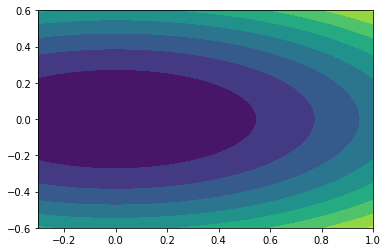In :
Gradf = lambda x : np.array([x,eta*x])


The step size should satisfy $\tau_k < 2/\eta$. We use here a constant step size.

In :
tau = 1.6/eta


Initial point for the descent.

In :
x0 = np.array( [.9,.3] )


Exercice 1:

Perform the gradient descent using a fixed step size $\tau_k=\tau$. Display the decay of the energy $f(x^{(k)})$ through the iteration. Save the iterates so that X(:,k) corresponds to $x^{(k)}$.

In :
run -i nt_solutions/optim_1_gradient_descent/exo1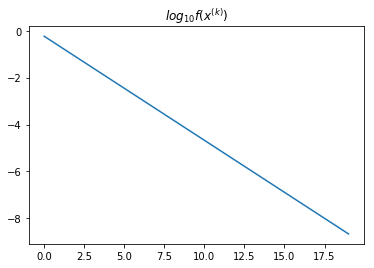Display the iterations.

In :
plt.contourf(tx,ty,F.transpose(),10);
plt.plot(X[0,:], X[1,:], 'k.-');
plt.axis('equal');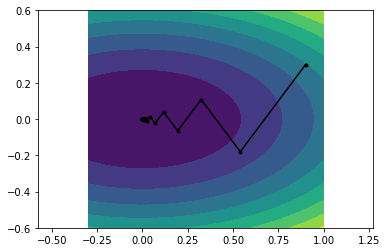Exercice 2:

Display the iteration for several different step sizes.

In :
run -i nt_solutions/optim_1_gradient_descent/exo2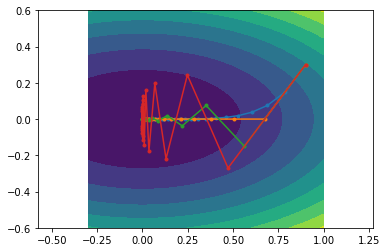Exercice 3:

Implement the optimal step size selection (exact linesearch).

In :
run -i nt_solutions/optim_1_gradient_descent/exo3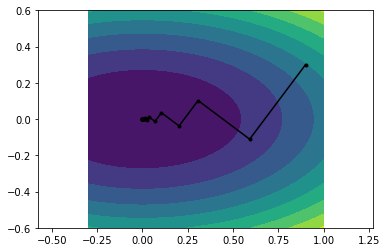## Gradient and Divergence of Images¶

Local differential operators like gradient, divergence and laplacian are the building blocks for variational image processing.

Load an image $x_0 \in \RR^N$ of $N=n \times n$ pixels.

In :
#from __future__ import division
from nt_toolbox.general import *
from nt_toolbox.signal import *
%pylab inline

Populating the interactive namespace from numpy and matplotlib

/Users/gpeyre/anaconda/envs/p34/lib/python3.4/site-packages/IPython/core/magics/pylab.py:161: UserWarning: pylab import has clobbered these variables: ['pylab', 'f']
%matplotlib prevents importing * from pylab and numpy
"\n%matplotlib prevents importing * from pylab and numpy"

In :
n = 256
name = 'nt_toolbox/data/lena.png'
x0 = load_image(name, n)

/Users/gpeyre/anaconda/envs/p34/lib/python3.4/site-packages/skimage/transform/_warps.py:84: UserWarning: The default mode, 'constant', will be changed to 'reflect' in skimage 0.15.
warn("The default mode, 'constant', will be changed to 'reflect' in "


Display it.

In :
imageplot(x0)For a continuous function $g$, the gradient reads $$\nabla g(s) = \pa{ \pd{g(s)}{s_1}, \pd{g(s)}{s_2} } \in \RR^2.$$ (note that here, the variable $d$ denotes the 2-D spacial position).

We discretize this differential operator on a discrete image $x \in \RR^N$ using first order finite differences. $$(\nabla x)_i = ( x_{i_1,i_2}-x_{i_1-1,i_2}, x_{i_1,i_2}-x_{i_1,i_2-1} ) \in \RR^2.$$ Note that for simplity we use periodic boundary conditions.

Compute its gradient, using finite differences.

In :
def grad(x):
hdiff = x - x[r_[len(x)-1,:len(x)-1],:]
vdiff = x - x[:,r_[len(x)-1,:len(x)-1]]
return concatenate((hdiff[...,newaxis],vdiff[...,newaxis]),axis=2)


One thus has $\nabla : \RR^N \mapsto \RR^{N \times 2}.$

In :
v = grad(x0)


One can display each of its components.

In :
imageplot(v[:,:,0], 'd/dx', (1,2,1))
imageplot(v[:,:,1], 'd/dy', (1,2,2))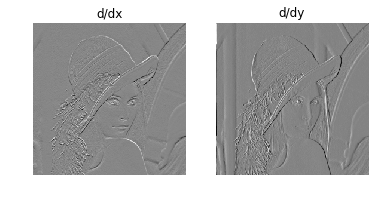One can display its magnitude $\norm{(\nabla x)_i}$, which is large near edges.

In :
imageplot(sqrt(sum(v * v,axis=2)))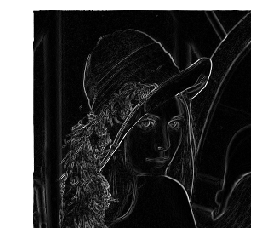The divergence operator maps vector field to images. For continuous vector fields (v(s) \in \RR^2), it is defined as $$\text{div}(v)(s) = \pd{v_1(s)}{s_1} + \pd{v_2(s)}{s_2} \in \RR.$$ (note that here, the variable (s) denotes the 2-D spacial position). It is minus the adjoint of the gadient, i.e. $\text{div} = - \nabla^*$.

It is discretized, for $v=(v^1,v^2)$ as $$\text{div}(v)_i = v^1_{i_1+1,i_2} - v^1_{i_1,i_2} + v^2_{i_1,i_2+1} - v^2_{i_1,i_2} .$$

In :
div = lambda x : x[r_[1:len(x),0],:,0] - x[:,:,0] + x[:,r_[1:len(x),0],1] - x[:,:,1]


The Laplacian operatore is defined as $\Delta=\text{div} \circ \nabla = -\nabla^* \circ \nabla$. It is thus a negative symmetric operator.

In :
delta = lambda x : div(grad(x))


Display $\Delta x_0$.

In :
imageplot(delta(x0))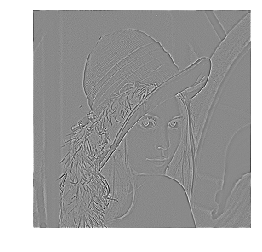Check that the relation $\norm{\nabla x} = - \dotp{\Delta x}{x}.$

In :
print('Should be 0:\n', (grad(x0) * grad(x0)).sum() + (delta(x0) * x0).sum() )

Should be 0:
-1.13686837722e-13


## Gradient Descent in Image Processing¶

We consider now the problem of denoising an image $y \in \RR^d$ where $d = n \times n$ is the number of pixels ($n$ being the number of rows/columns in the image).

Add noise to the original image, to simulate a noisy image.

In :
sigma = 0.1
y = x0 + sigma * randn(n,n)


Display the noisy image $y$.

In :
imageplot(clamp(y))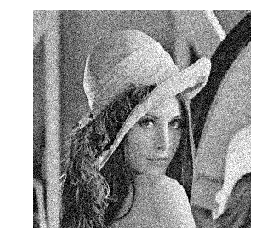Denoising is obtained by minimizing the following functional $$\umin{x \in \RR^d} f(x) = \frac{1}{2} \norm{y-x}^2 + \la J_\epsilon(x)$$ where $J_\epsilon(x)$ is a smoothed total variation of the image. $$J_\epsilon(x) = \sum_i \norm{ (G x)_i }_{\epsilon}$$ where $(Gx)_i \in \RR^2$ is an approximation of the gradient of $x$ at pixel $i$ and for $u \in \RR^2$, we use the following smoothing of the $L^2$ norm in $\RR^2$ $$\norm{u}_\epsilon = \sqrt{ \epsilon^2 + \norm{u}^2 },$$ for a small value of $\epsilon>0$.

The gradient of the functional read $$\nabla f(x) = x-y + \lambda \nabla J_\epsilon(x)$$ where the gradient of the smoothed TV norm is $$\nabla J_\epsilon(x)_i = G^*( u ) \qwhereq u_i = \frac{ (G x)_i }{\norm{ (G x)_i }_\epsilon}$$ where $G^*$ is the adjoint operator of $G$ which corresponds to minus a discretized divergence

Value for $\lambda$.

In :
la = 0.3 / 5


Value for $\epsilon$.

In :
epsilon = 1e-3


TV norm.

In :
norm_eps = lambda u : sqrt(eps**2 + sum(u * u, axis=2))
J = lambda x : sum(sum(norm_eps(grad(x))))


Function $f$ to minimize.

In :
f = lambda x : 1/2 * norm(x-y)**2 + la * J(x)


Gradient of $J_\epsilon$. Note that |div| implement $-G^*$.

In :
normalize_vec = lambda u : u / tile(norm_eps(u)[...,newaxis], (1, 1, 2))
gradTV = lambda x : - divergence(normalize_vec(grad(x)))


Gradient of the functional.

In :
Gradf = lambda x : x - y + la * gradTV(x)


The step size should satisfy $$0 < \tau_k < \frac{2}{ 1 + 4 \lambda / \epsilon }.$$ Here we use a slightly larger step size, which still work in practice.

In :
tau = 1.8/( 1 + la*8/epsilon )
tau = tau*4


Exercice 3: Implement the gradient descent. Monitor the decay of $f$ through the iterations.

In :
## Insert your code here.


Display the resulting denoised image.

In :
#imageplot(clamp(x))


## Constrained Optimization Using Projected Gradient Descent¶

We consider a linear imaging operator $\Phi : x \mapsto \Phi(x)$ that maps high resolution images to low dimensional observations. Here we consider a pixel masking operator, that is diagonal over the spacial domain.

To emphasis the effect of the TV functional, we use a simple geometric image.

In :
n = 64
t = linspace(-1,1,n)
[Y,X] = meshgrid(t,t)
x0 = (maximum( abs(X),abs(Y) ) < radius) * 1.0


We consider here the inpainting problem. This simply corresponds to a masking operator. Here we remove the central part of the image.

In :
a = 4
Lambda = ones((n,n))
Lambda[n/2-a:n/2+a,:] = 0

nt_solutions/optim_1_gradient_descent/exo3.py:3: VisibleDeprecationWarning: using a non-integer number instead of an integer will result in an error in the future
x = x0


Masking operator $\Phi$. Note that it is symmetric, i.e. $\Phi^*=\Phi$.

In :
Phi  = lambda x : x * Lambda
PhiS = Phi


Noiseless observations $y=\Phi x_0$.

In :
y = Phi(x0)


Display.

In :
imageplot(x0, 'Original', (1,2,1))
imageplot(y, 'Damaged', (1,2,2))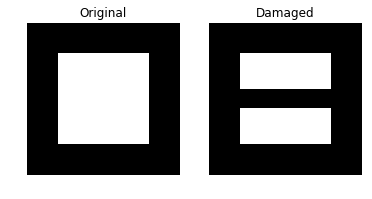We want to solve the noiseless inverse problem $y=\Phi f$ using a total variation regularization: $$\umin{ y=\Phi x } J_\epsilon(x).$$ We use the following projected gradient descent $$x^{(k+1)} = \text{Proj}_{\Hh}( x^{(k)} - \tau_k \nabla J_{\epsilon}(x^{(k)}) )$$ where $\text{Proj}_{\Hh}$ is the orthogonal projection on the set of linear constraint $\Phi x = y$, and is easy to compute for inpainting

In :
ProjH = lambda x : x + PhiS( y - Phi(x) )


Exercice 4: Display the evolution of the inpainting process.

In :
## Insert your code here.


Exercice 5: Try with several values of $\epsilon$.

In :
## Insert your code here.